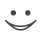# This flashcard is just one of a free flashcard set. See all flashcards!

108
Which of the following is true?
A.The optimal hedge ratio is the slope of the best fit line when the spot price (on the y-axis) is regressed against the futures price (on the x-axis).
B.The optimal hedge ratio is the slope of the best fit line when the futures price (on the y-axis) is regressed against the spot price (on the x-axis).
C.The optimal hedge ratio is the slope of the best fit line when the change in the spot price (on the y-axis) is regressed against the change in the futures price (on the x-axis).
D.The optimal hedge ratio is the slope of the best fit line when the change in the futures price (on the y-axis) is regressed against the change in the spot price (on the x-axis).